# Azure Terraform（四）状态文件存储

我们都知道在执行部署计划之后，当前目录中就产生了名叫 “terraform.states” 的 Terraform 的状态文件，该文件中记录了已部署资源的状态。默认情况下，在执行部署计划后，Terraform 的状态文件会存储在本地，但是这样往往就造成一些弊端：

（1）不适用团队之间协助，就好比在数据库中对同一条数据进行操作时，就会引起异常

（2）状态文件中包含一些机密信息，会造成一定的机密泄露

（3）如果不慎将本地的状态文件删除掉的话，已执行部署计划的资源的管理将很难在通过 Terraform 进行管理

--------------------Azure Terraform 系列--------------------

1，Azure Terraform（一）入门简介
2，Azure Terraform（二）语法详解
3，Azure Terraform（三）部署 Web 应用程序
4，Azure Terraform（四）状态文件存储

1，创建状态文件存储账户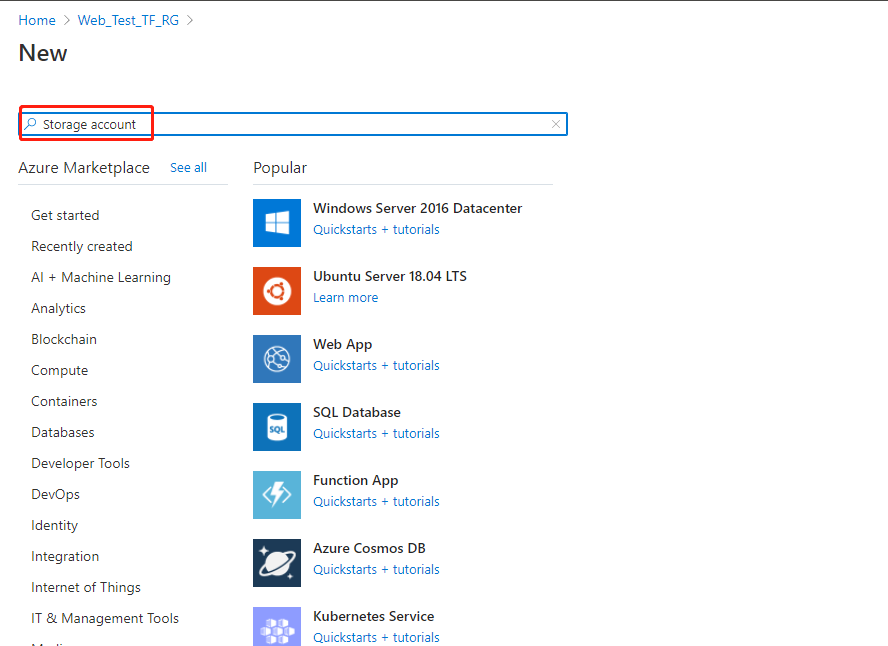Resource group 选择：”Web_Test_TF_RG“

Storage account name：”cnbateterraformstorage“

Location：”(Asia Pacific) East Asia“

Performance：”Standard“

Account kind （账户类型）选择：”BlobStorage“

Replication （复制）选择：”Locally-redundant storage（LRS）“ (本地冗余存储（LRS）)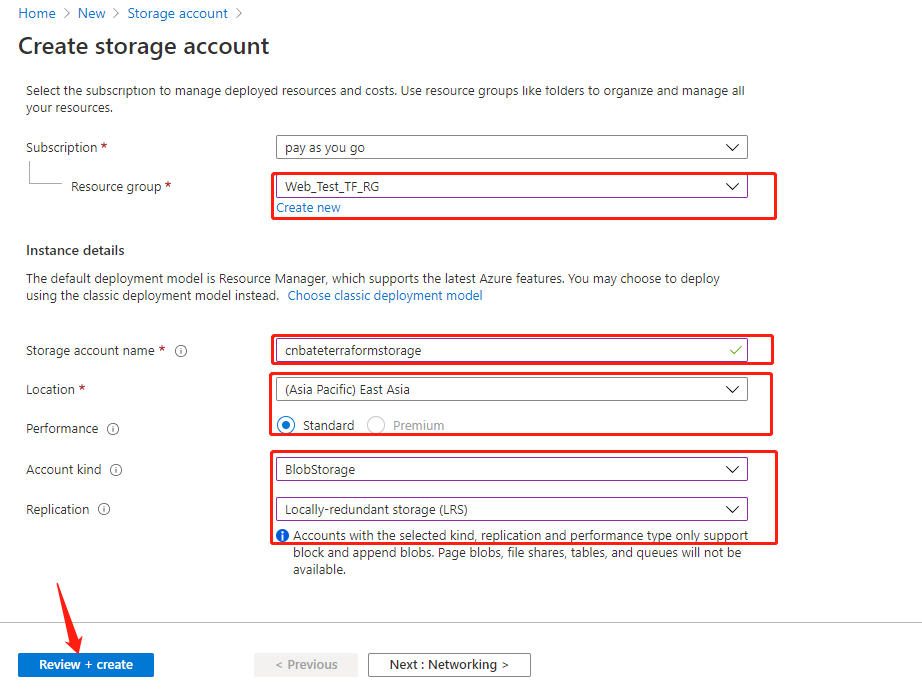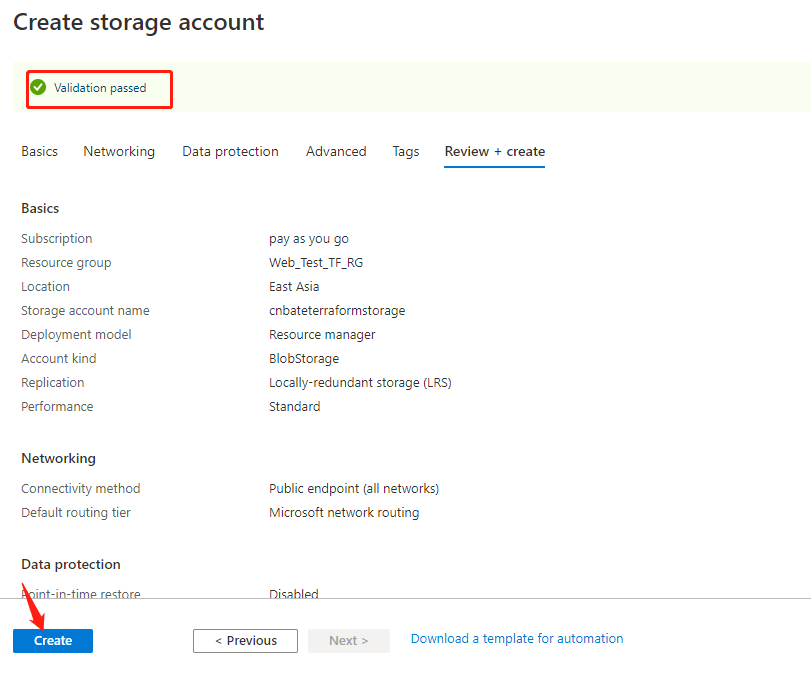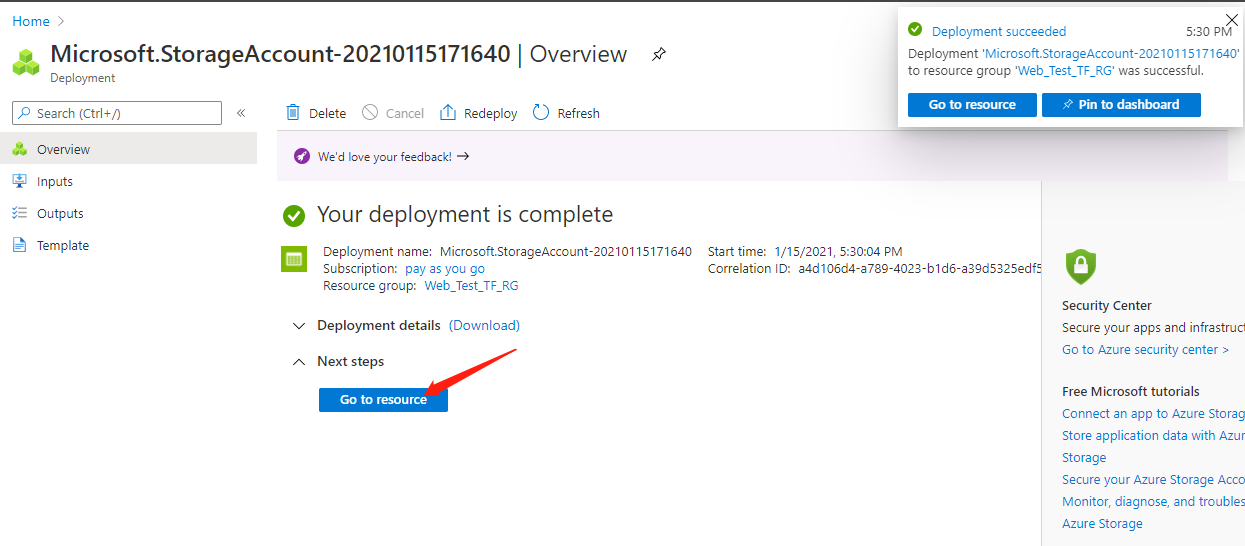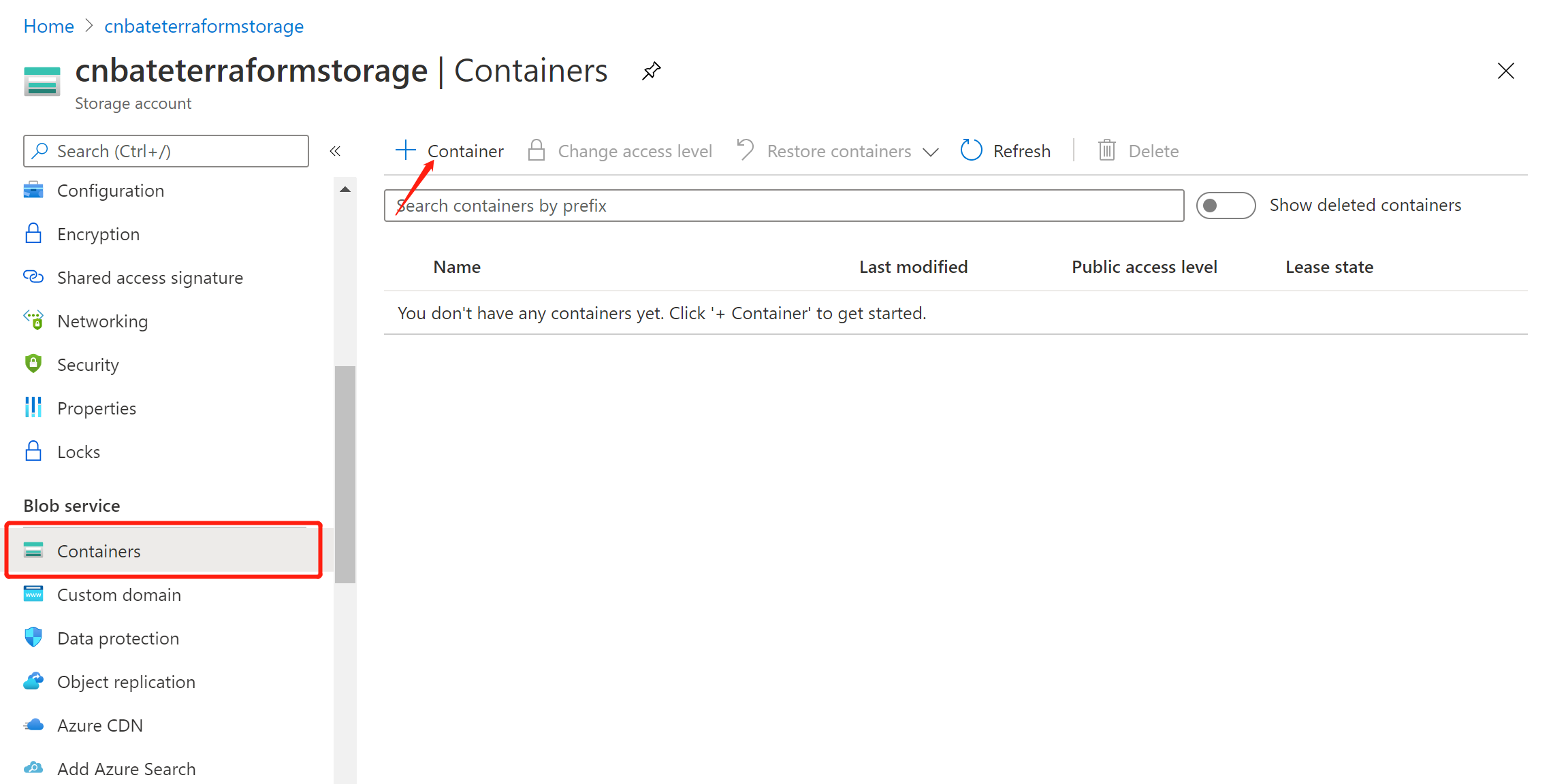Name："terraform-state"

Public access level：“Private（no anonymous access）”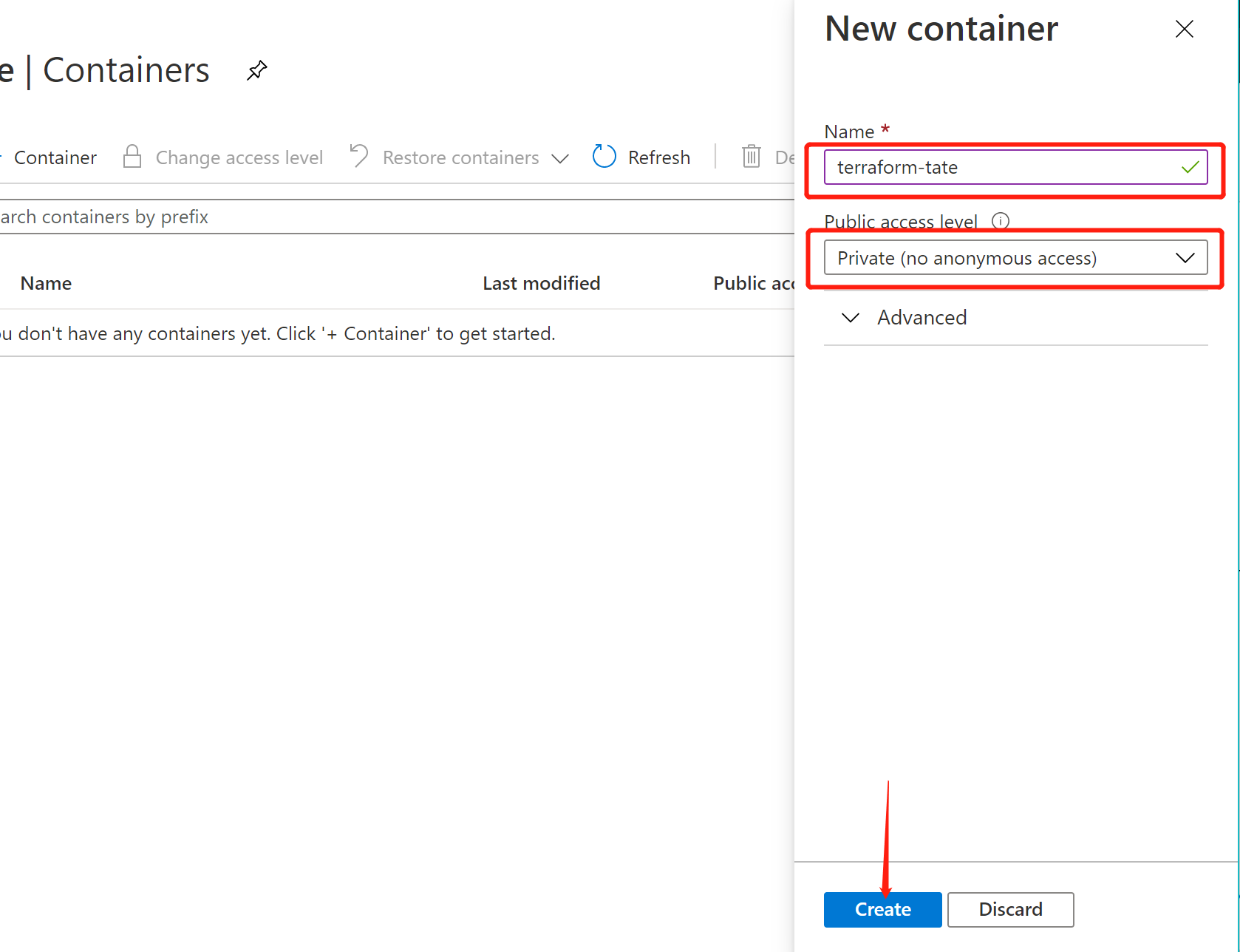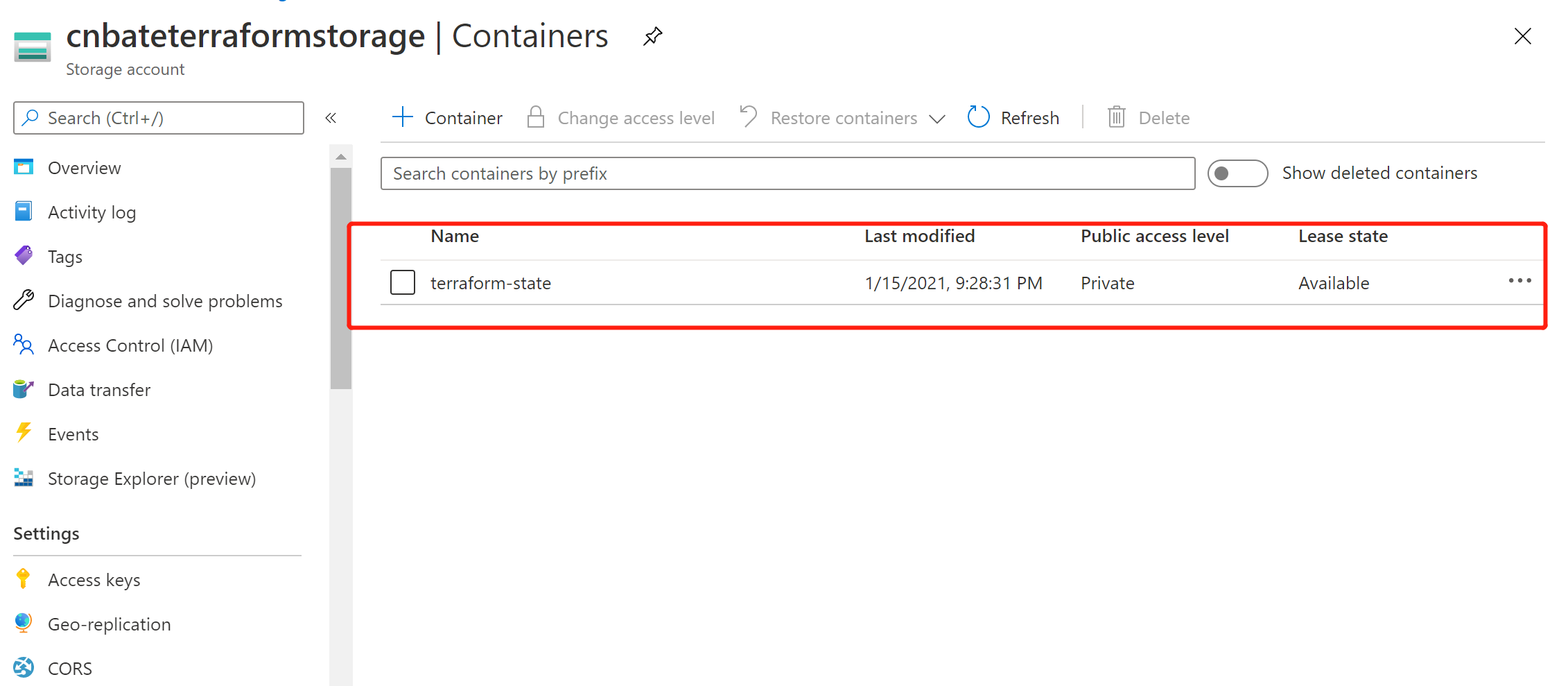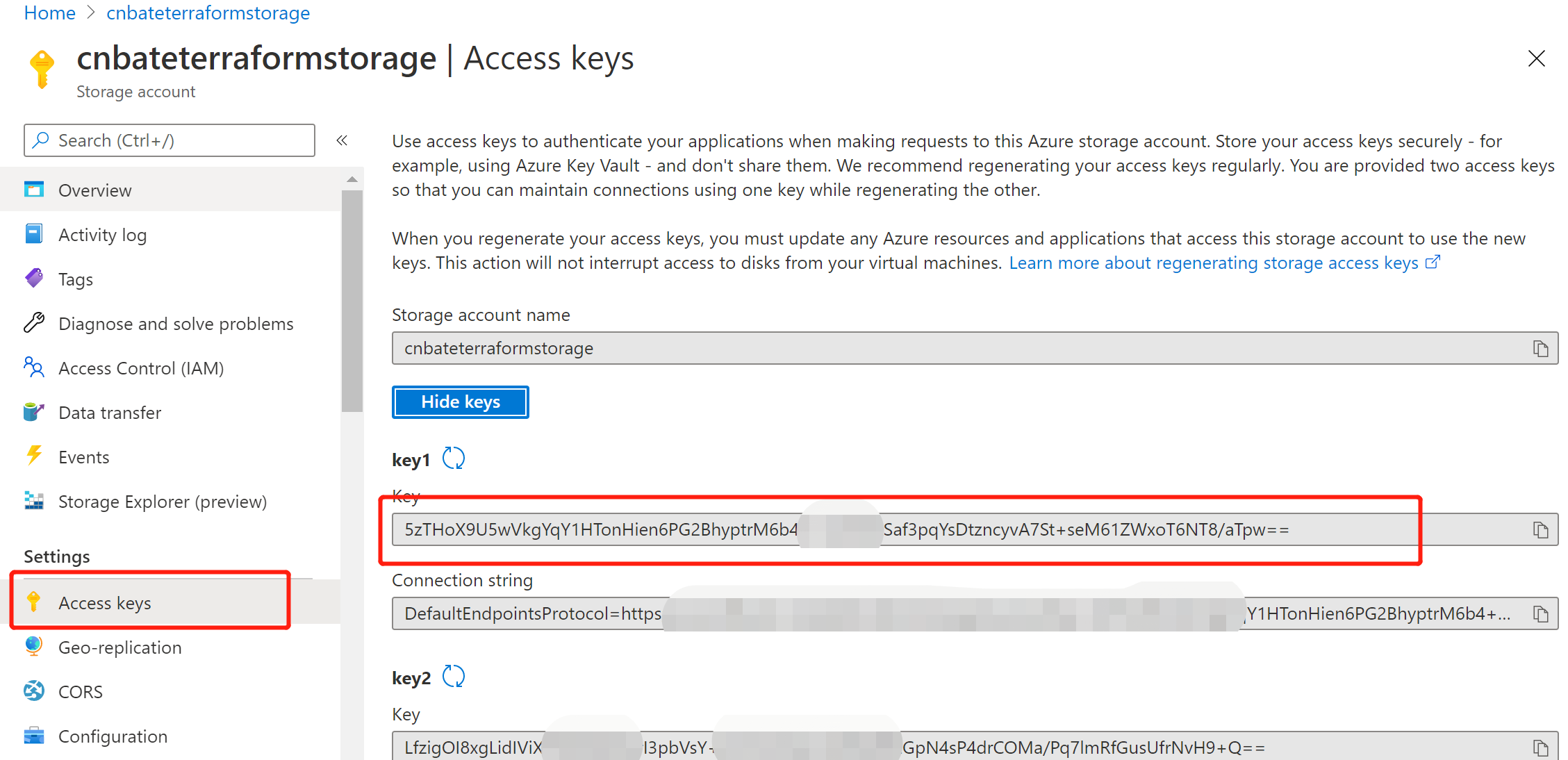2，创建 Azure Key Vault（密钥保管库）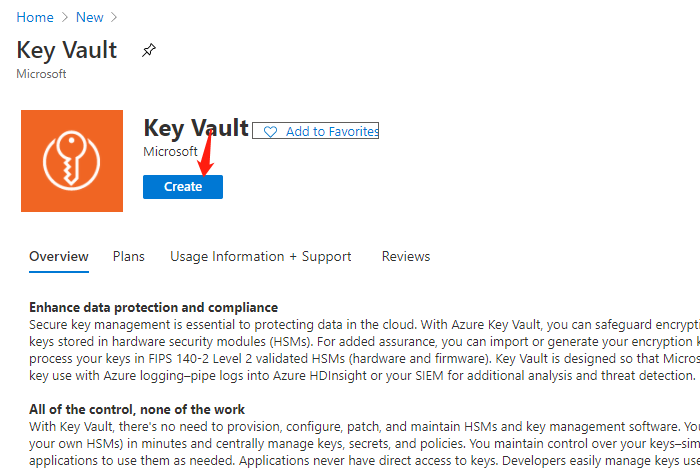Resource group 选择：”Web_Test_TF_RG“

Key vault name：”cnbate-terraform-kv“

Region：”East Asia“

Pricing tier：”Standard“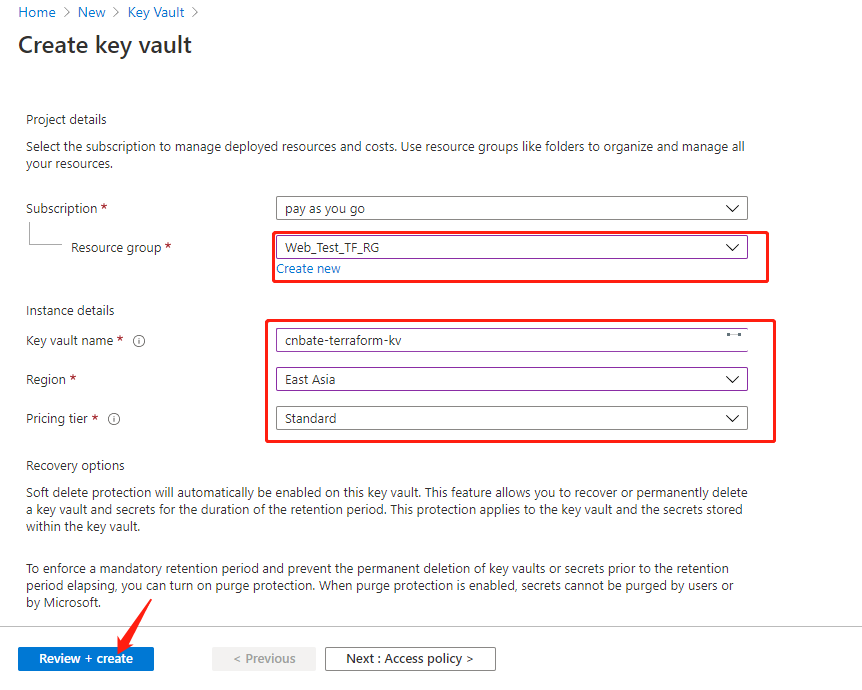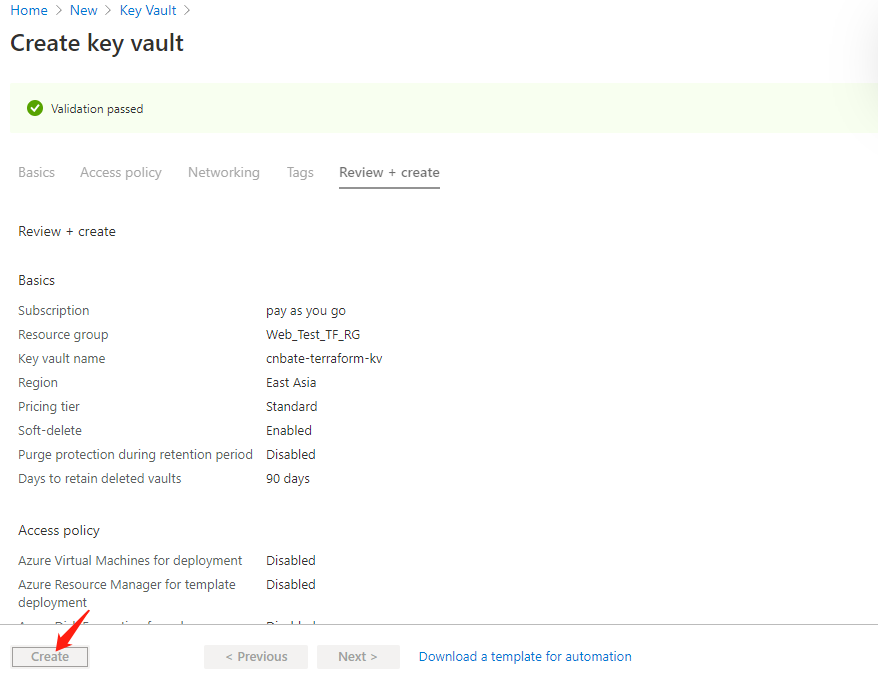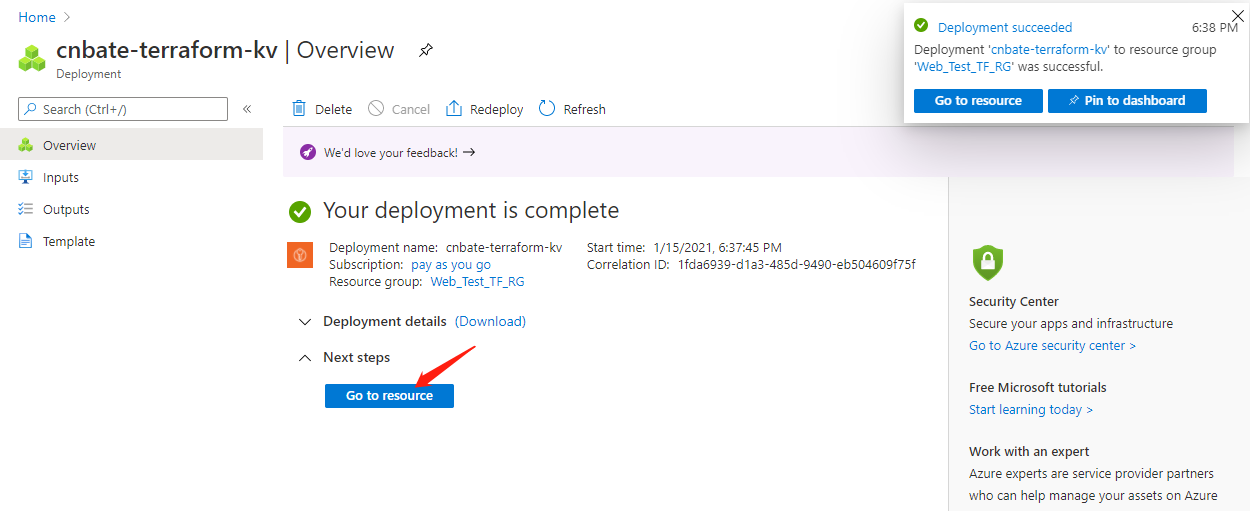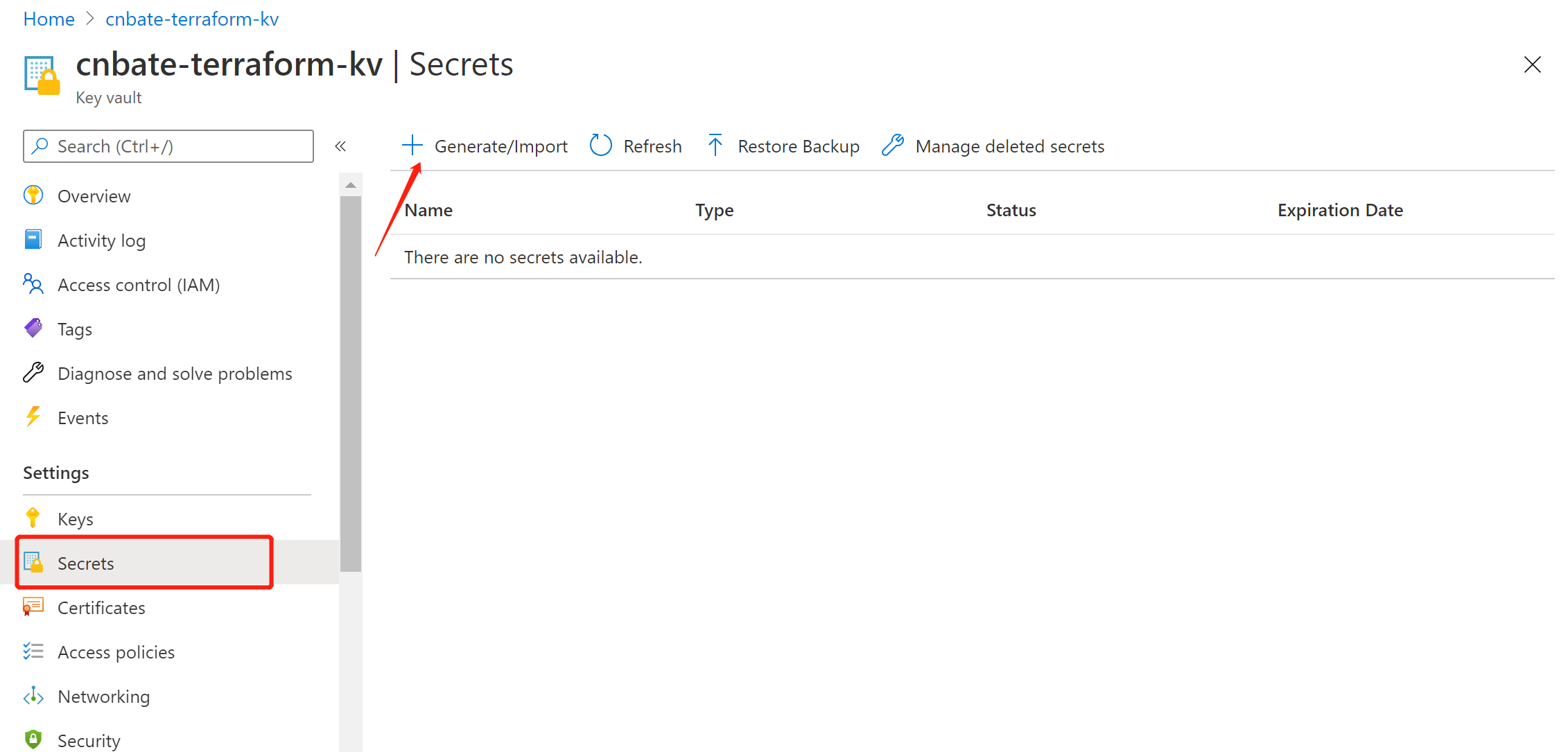Upload options：“Manual”（手动）

Name：“terraform-stste-storage-key”

Value：复制粘贴刚刚的存储账户访问密钥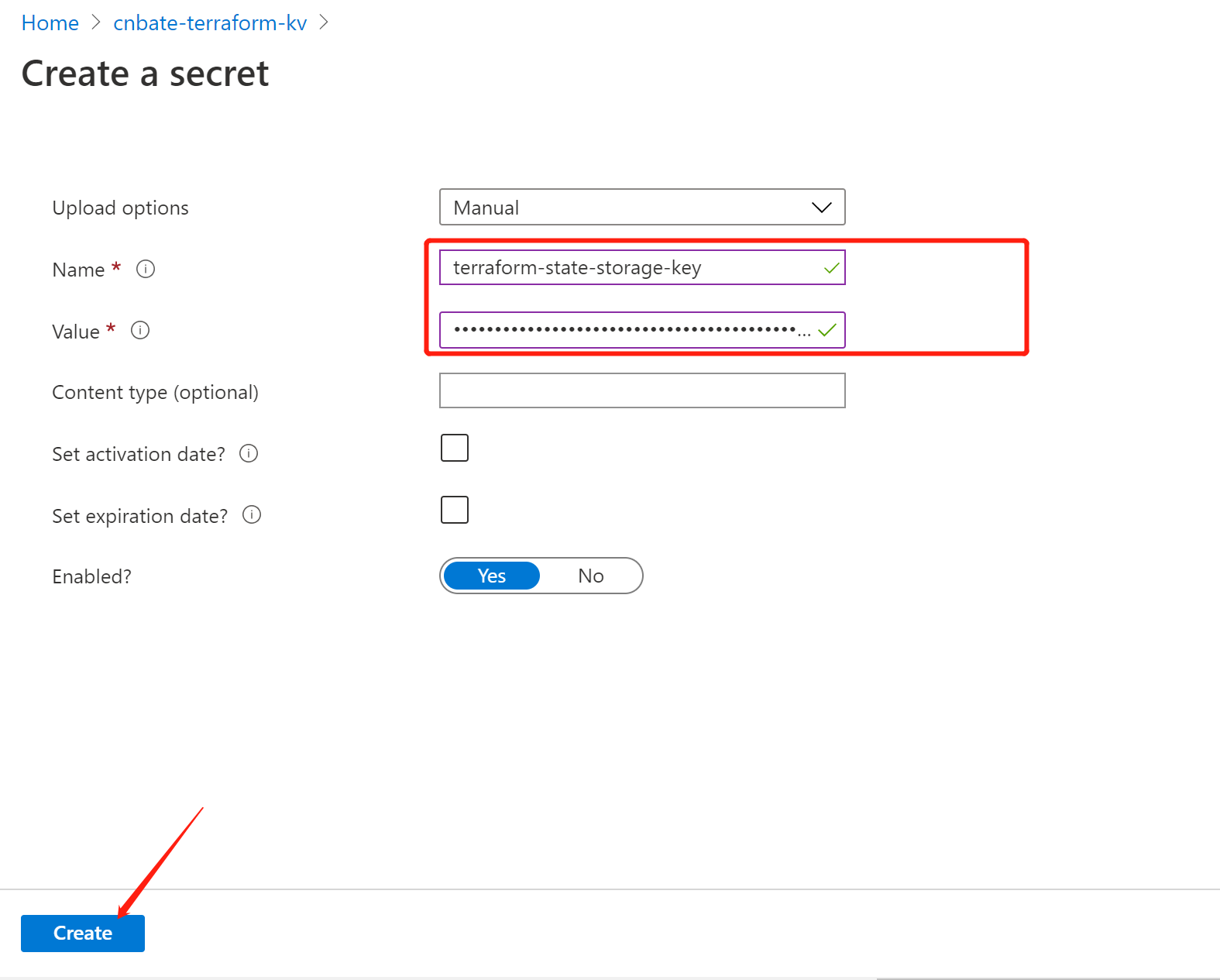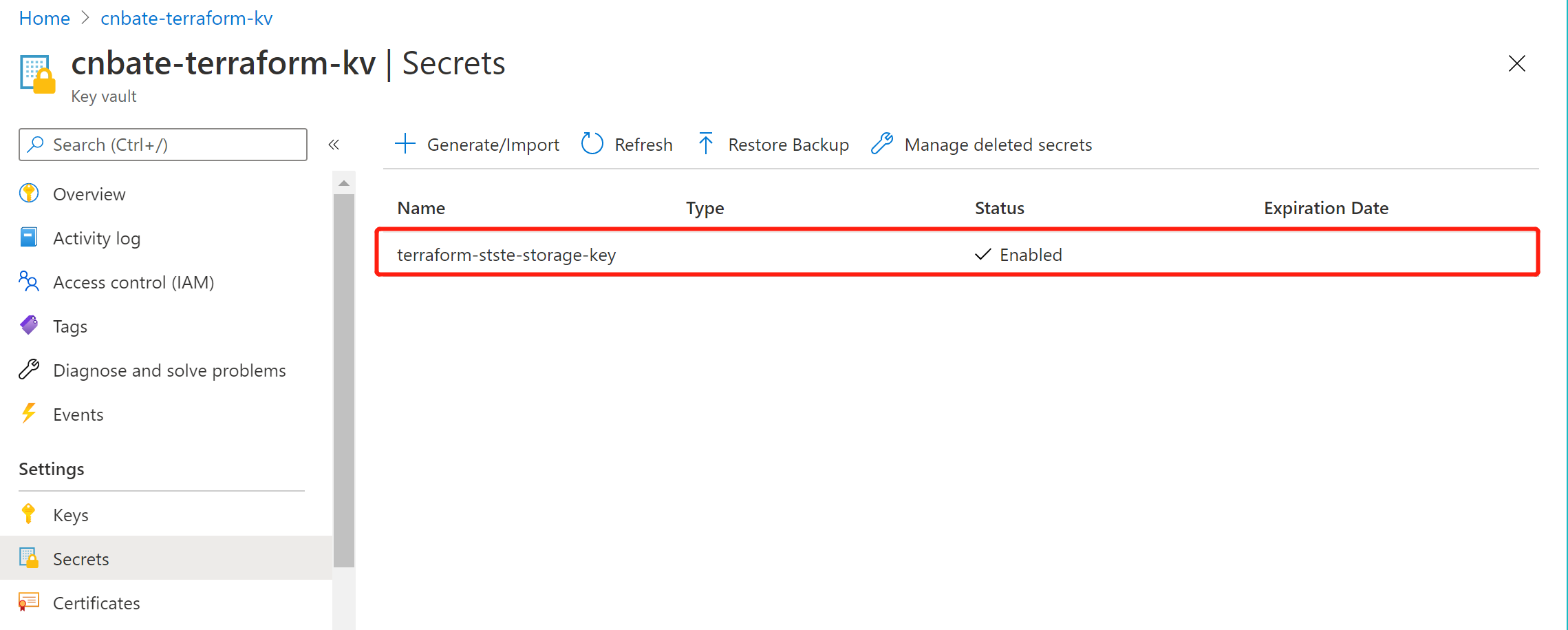3，配置 Terraform 后端，并且测试远程 tf 状态

Terraform 需要配置后端，需要以下参数

（1）storage_account_name ：Azure 存储账户名称

terraform init -backend-config="access_key=\$(az keyvault secret show --name terraform-stste-storage-key --vault-name cnbate-terraform-kv --query value -o tsv)"

（2）container_name：容器名称

（3）key：存储状态文件的名称

（4）access_key：存储账户访问密钥

terraform {
backend "azurerm" {
storage_account_name = "cnbateterraformstorage"
container_name = "terraform-state"
key = "cnbate.terraform.stats"
}
}

3.1，初始化 Terraform 代码

terraform init -backend-config="access_key=\$(az keyvault secret show --name terraform-stste-storage-key --vault-name cnbate-terraform-kv --query value -o tsv)"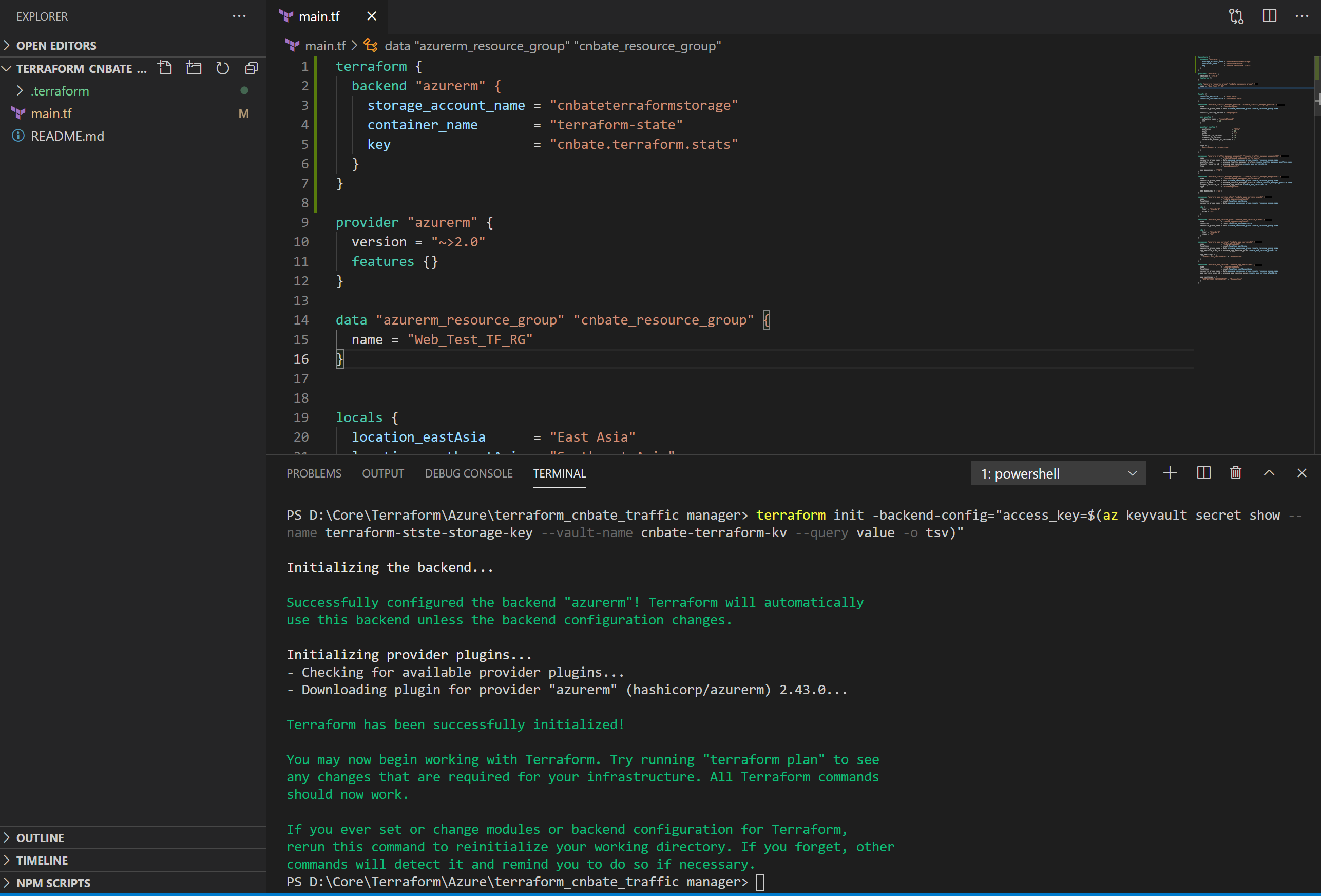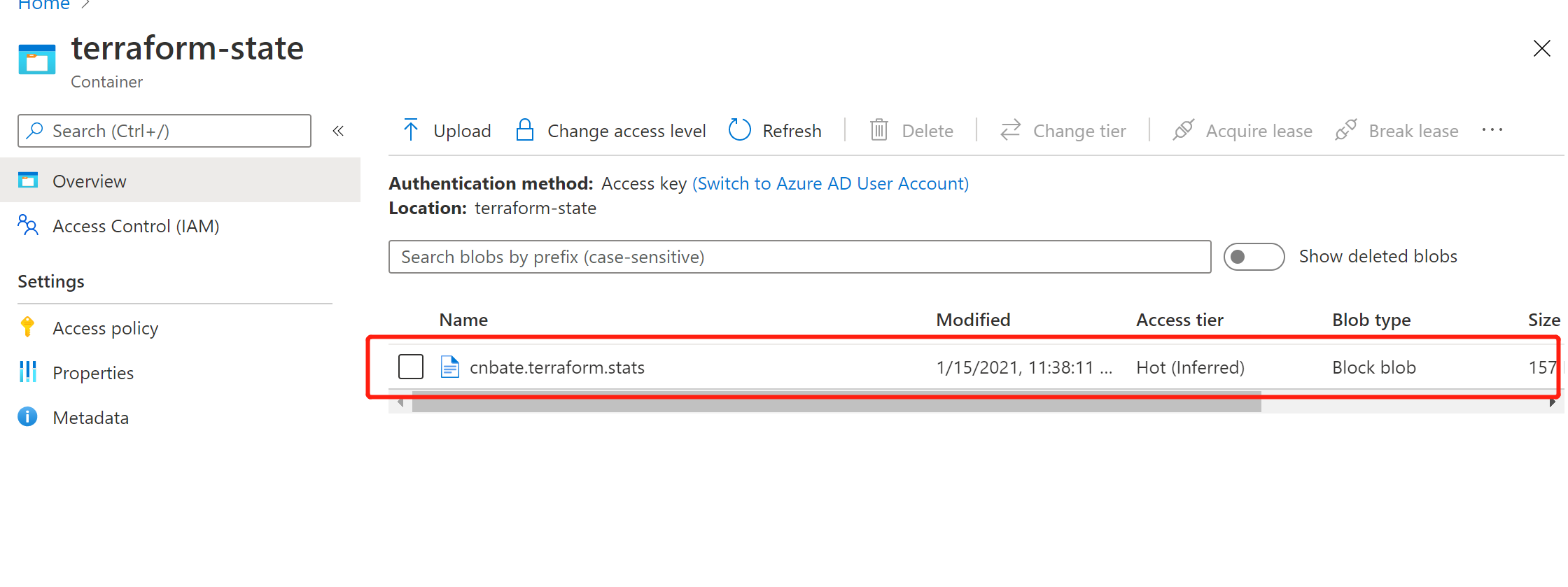LEASE STATUS：解锁状态

LEASE STATE：可用状态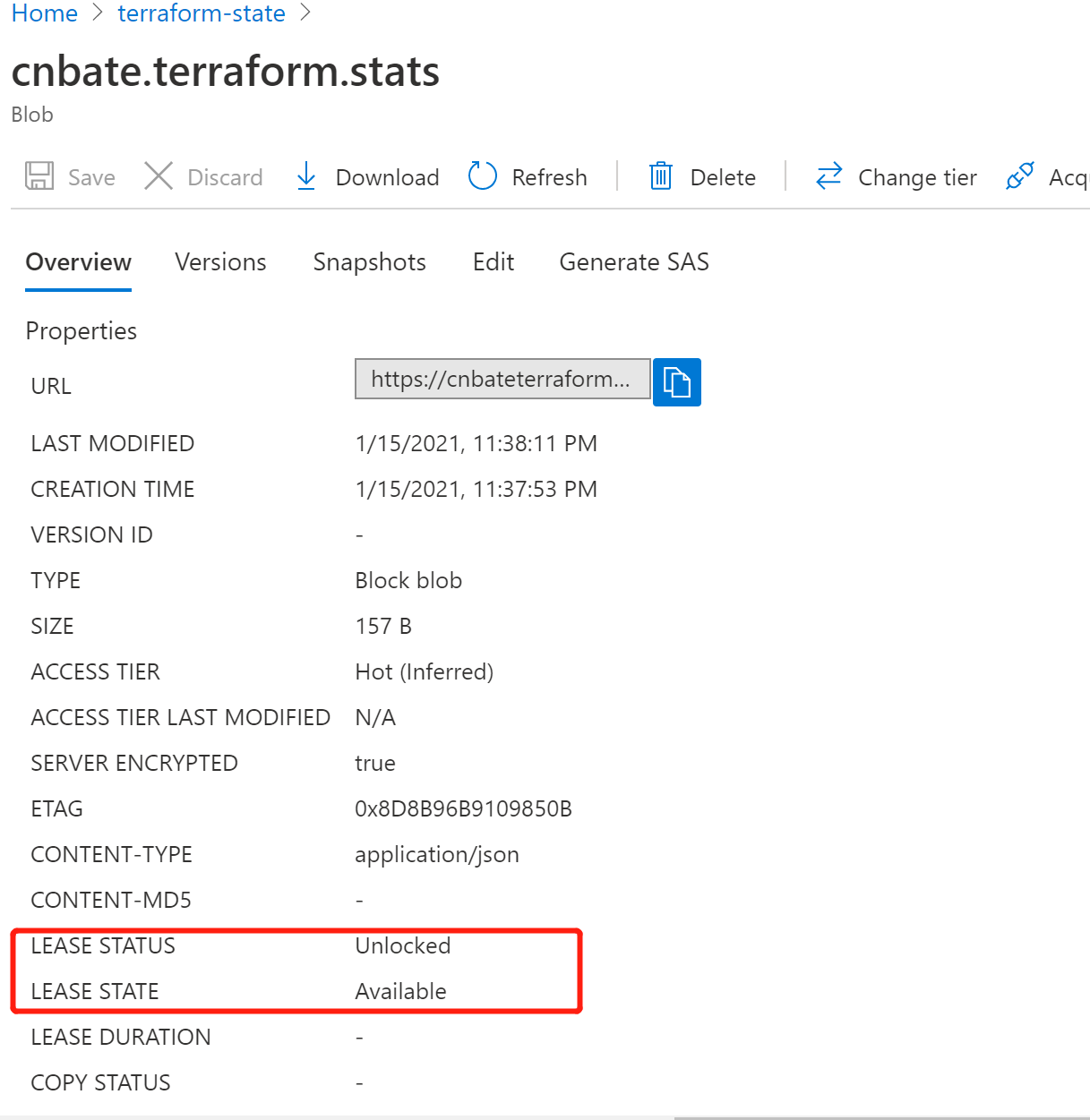3.2，生成执行计划

terraform plan

PS D:CoreTerraformAzure erraform_cnbate_traffic manager> terraform plan
Acquiring state lock. This may take a few moments...
Refreshing Terraform state in-memory prior to plan...
The refreshed state will be used to calculate this plan, but will not be
persisted to local or remote state storage.
data.azurerm_resource_group.cnbate_resource_group: Refreshing state...
------------------------------------------------------------------------
An execution plan has been generated and is shown below.
Resource actions are indicated with the following symbols:
+ create
Terraform will perform the following actions:
# azurerm_app_service.cnbate_app_service01 will be created
+ resource "azurerm_app_service" "cnbate_app_service01" {
+ app_service_plan_id = (known after apply)
+ app_settings = {
+ "ASPNETCORE_ENVIRONMENT" = "Production"
}
+ client_affinity_enabled = false
+ client_cert_enabled = false
+ custom_domain_verification_id = (known after apply)
+ default_site_hostname = (known after apply)
+ enabled = true
+ https_only = false
+ id = (known after apply)
+ location = "eastasia"
+ name = "CnBateBlogWeb01"
+ outbound_ip_address_list = (known after apply)
+ outbound_ip_addresses = (known after apply)
+ possible_outbound_ip_address_list = (known after apply)
+ possible_outbound_ip_addresses = (known after apply)
+ resource_group_name = "Web_Test_TF_RG"
+ site_credential = (known after apply)
+ auth_settings {
+ additional_login_params = (known after apply)
+ allowed_external_redirect_urls = (known after apply)
+ default_provider = (known after apply)
+ enabled = (known after apply)
+ issuer = (known after apply)
+ runtime_version = (known after apply)
+ token_refresh_extension_hours = (known after apply)
+ token_store_enabled = (known after apply)
+ unauthenticated_client_action = (known after apply)
+ active_directory {
+ allowed_audiences = (known after apply)
+ client_id = (known after apply)
+ client_secret = (sensitive value)
}
+ facebook {
+ app_id = (known after apply)
+ app_secret = (sensitive value)
+ oauth_scopes = (known after apply)
}
+ google {
+ client_id = (known after apply)
+ client_secret = (sensitive value)
+ oauth_scopes = (known after apply)
}
+ microsoft {
+ client_id = (known after apply)
+ client_secret = (sensitive value)
+ oauth_scopes = (known after apply)
}
+ twitter {
+ consumer_key = (known after apply)
+ consumer_secret = (sensitive value)
}
}
+ connection_string {
+ name = (known after apply)
+ type = (known after apply)
+ value = (sensitive value)
}
+ identity {
+ identity_ids = (known after apply)
+ principal_id = (known after apply)
+ tenant_id = (known after apply)
+ type = (known after apply)
}
+ logs {
+ detailed_error_messages_enabled = (known after apply)
+ failed_request_tracing_enabled = (known after apply)
+ application_logs {
+ file_system_level = (known after apply)
+ azure_blob_storage {
+ level = (known after apply)
+ retention_in_days = (known after apply)
+ sas_url = (sensitive value)
}
}
+ http_logs {
+ azure_blob_storage {
+ retention_in_days = (known after apply)
+ sas_url = (sensitive value)
}
+ file_system {
+ retention_in_days = (known after apply)
+ retention_in_mb = (known after apply)
}
}
}
+ site_config {
+ always_on = (known after apply)
+ app_command_line = (known after apply)
+ auto_swap_slot_name = (known after apply)
+ default_documents = (known after apply)
+ dotnet_framework_version = (known after apply)
+ ftps_state = (known after apply)
+ health_check_path = (known after apply)
+ http2_enabled = (known after apply)
+ ip_restriction = (known after apply)
+ java_container = (known after apply)
+ java_container_version = (known after apply)
+ java_version = (known after apply)
+ linux_fx_version = (known after apply)
+ local_mysql_enabled = (known after apply)
+ managed_pipeline_mode = (known after apply)
+ min_tls_version = (known after apply)
+ php_version = (known after apply)
+ python_version = (known after apply)
+ remote_debugging_enabled = (known after apply)
+ remote_debugging_version = (known after apply)
+ scm_ip_restriction = (known after apply)
+ scm_type = (known after apply)
+ scm_use_main_ip_restriction = (known after apply)
+ use_32_bit_worker_process = (known after apply)
+ websockets_enabled = (known after apply)
+ windows_fx_version = (known after apply)
+ cors {
+ allowed_origins = (known after apply)
+ support_credentials = (known after apply)
}
}
+ source_control {
+ branch = (known after apply)
+ manual_integration = (known after apply)
+ repo_url = (known after apply)
+ rollback_enabled = (known after apply)
+ use_mercurial = (known after apply)
}
+ storage_account {
+ access_key = (sensitive value)
+ account_name = (known after apply)
+ mount_path = (known after apply)
+ name = (known after apply)
+ share_name = (known after apply)
+ type = (known after apply)
}
}
# azurerm_app_service.cnbate_app_service02 will be created
+ resource "azurerm_app_service" "cnbate_app_service02" {
+ app_service_plan_id = (known after apply)
+ app_settings = {
+ "ASPNETCORE_ENVIRONMENT" = "Production"
}
+ client_affinity_enabled = false
+ client_cert_enabled = false
+ custom_domain_verification_id = (known after apply)
+ default_site_hostname = (known after apply)
+ enabled = true
+ https_only = false
+ id = (known after apply)
+ location = "southeastasia"
+ name = "CnBateBlogWeb02"
+ outbound_ip_address_list = (known after apply)
+ outbound_ip_addresses = (known after apply)
+ possible_outbound_ip_address_list = (known after apply)
+ possible_outbound_ip_addresses = (known after apply)
+ resource_group_name = "Web_Test_TF_RG"
+ site_credential = (known after apply)
+ auth_settings {
+ additional_login_params = (known after apply)
+ allowed_external_redirect_urls = (known after apply)
+ default_provider = (known after apply)
+ enabled = (known after apply)
+ issuer = (known after apply)
+ runtime_version = (known after apply)
+ token_refresh_extension_hours = (known after apply)
+ token_store_enabled = (known after apply)
+ unauthenticated_client_action = (known after apply)
+ active_directory {
+ allowed_audiences = (known after apply)
+ client_id = (known after apply)
+ client_secret = (sensitive value)
}
+ facebook {
+ app_id = (known after apply)
+ app_secret = (sensitive value)
+ oauth_scopes = (known after apply)
}
+ google {
+ client_id = (known after apply)
+ client_secret = (sensitive value)
+ oauth_scopes = (known after apply)
}
+ microsoft {
+ client_id = (known after apply)
+ client_secret = (sensitive value)
+ oauth_scopes = (known after apply)
}
+ twitter {
+ consumer_key = (known after apply)
+ consumer_secret = (sensitive value)
}
}
+ connection_string {
+ name = (known after apply)
+ type = (known after apply)
+ value = (sensitive value)
}
+ identity {
+ identity_ids = (known after apply)
+ principal_id = (known after apply)
+ tenant_id = (known after apply)
+ type = (known after apply)
}
+ logs {
+ detailed_error_messages_enabled = (known after apply)
+ failed_request_tracing_enabled = (known after apply)
+ application_logs {
+ file_system_level = (known after apply)
+ azure_blob_storage {
+ level = (known after apply)
+ retention_in_days = (known after apply)
+ sas_url = (sensitive value)
}
}
+ http_logs {
+ azure_blob_storage {
+ retention_in_days = (known after apply)
+ sas_url = (sensitive value)
}
+ file_system {
+ retention_in_days = (known after apply)
+ retention_in_mb = (known after apply)
}
}
}
+ site_config {
+ always_on = (known after apply)
+ app_command_line = (known after apply)
+ auto_swap_slot_name = (known after apply)
+ default_documents = (known after apply)
+ dotnet_framework_version = (known after apply)
+ ftps_state = (known after apply)
+ health_check_path = (known after apply)
+ http2_enabled = (known after apply)
+ ip_restriction = (known after apply)
+ java_container = (known after apply)
+ java_container_version = (known after apply)
+ java_version = (known after apply)
+ linux_fx_version = (known after apply)
+ local_mysql_enabled = (known after apply)
+ managed_pipeline_mode = (known after apply)
+ min_tls_version = (known after apply)
+ php_version = (known after apply)
+ python_version = (known after apply)
+ remote_debugging_enabled = (known after apply)
+ remote_debugging_version = (known after apply)
+ scm_ip_restriction = (known after apply)
+ scm_type = (known after apply)
+ scm_use_main_ip_restriction = (known after apply)
+ use_32_bit_worker_process = (known after apply)
+ websockets_enabled = (known after apply)
+ windows_fx_version = (known after apply)
+ cors {
+ allowed_origins = (known after apply)
+ support_credentials = (known after apply)
}
}
+ source_control {
+ branch = (known after apply)
+ manual_integration = (known after apply)
+ repo_url = (known after apply)
+ rollback_enabled = (known after apply)
+ use_mercurial = (known after apply)
}
+ storage_account {
+ access_key = (sensitive value)
+ account_name = (known after apply)
+ mount_path = (known after apply)
+ name = (known after apply)
+ share_name = (known after apply)
+ type = (known after apply)
}
}
# azurerm_app_service_plan.cnbate_app_service_plan01 will be created
+ resource "azurerm_app_service_plan" "cnbate_app_service_plan01" {
+ id = (known after apply)
+ kind = "Windows"
+ location = "eastasia"
+ maximum_elastic_worker_count = (known after apply)
+ maximum_number_of_workers = (known after apply)
+ name = "cnbate_appserviceplan01"
+ resource_group_name = "Web_Test_TF_RG"
+ sku {
+ capacity = (known after apply)
+ size = "S1"
+ tier = "Standard"
}
}
# azurerm_app_service_plan.cnbate_app_service_plan02 will be created
+ resource "azurerm_app_service_plan" "cnbate_app_service_plan02" {
+ id = (known after apply)
+ kind = "Windows"
+ location = "southeastasia"
+ maximum_elastic_worker_count = (known after apply)
+ maximum_number_of_workers = (known after apply)
+ name = "cnbate_appserviceplan02"
+ resource_group_name = "Web_Test_TF_RG"
+ sku {
+ capacity = (known after apply)
+ size = "S1"
+ tier = "Standard"
}
}
# azurerm_traffic_manager_endpoint.cnbate_traffic_manager_endpoint01 will be created
+ resource "azurerm_traffic_manager_endpoint" "cnbate_traffic_manager_endpoint01" {
+ endpoint_location = (known after apply)
+ endpoint_monitor_status = (known after apply)
+ endpoint_status = (known after apply)
+ geo_mappings = [
+ "CN",
]
+ id = (known after apply)
+ name = "cnbateblogweb_webapp01_performance"
+ priority = (known after apply)
+ profile_name = "cnbateblogweb"
+ resource_group_name = "Web_Test_TF_RG"
+ target = (known after apply)
+ target_resource_id = (known after apply)
+ type = "azureEndpoints"
+ weight = (known after apply)
}
# azurerm_traffic_manager_endpoint.cnbate_traffic_manager_endpoint02 will be created
+ resource "azurerm_traffic_manager_endpoint" "cnbate_traffic_manager_endpoint02" {
+ endpoint_location = (known after apply)
+ endpoint_monitor_status = (known after apply)
+ endpoint_status = (known after apply)
+ geo_mappings = [
+ "SG",
]
+ id = (known after apply)
+ name = "cnbateblogweb_webapp02_performance"
+ priority = (known after apply)
+ profile_name = "cnbateblogweb"
+ resource_group_name = "Web_Test_TF_RG"
+ target = (known after apply)
+ target_resource_id = (known after apply)
+ type = "azureEndpoints"
+ weight = (known after apply)
}
# azurerm_traffic_manager_profile.cnbate_traffic_manager_profile will be created
+ resource "azurerm_traffic_manager_profile" "cnbate_traffic_manager_profile" {
+ fqdn = (known after apply)
+ id = (known after apply)
+ name = "cnbateblogweb"
+ profile_status = (known after apply)
+ resource_group_name = "Web_Test_TF_RG"
+ tags = {
+ "Environment" = "Production"
}
+ traffic_routing_method = "Geographic"
+ dns_config {
+ relative_name = "cnbateblogweb"
+ ttl = 60
}
+ monitor_config {
+ interval_in_seconds = 30
+ path = "/"
+ port = 80
+ protocol = "http"
+ timeout_in_seconds = 10
+ tolerated_number_of_failures = 3
}
}
Plan: 7 to add, 0 to change, 0 to destroy.
------------------------------------------------------------------------
Note: You didn"t specify an "-out" parameter to save this plan, so Terraform
can"t guarantee that exactly these actions will be performed if
"terraform apply" is subsequently run.
PS D:CoreTerraformAzure erraform_cnbate_traffic manager>

3.3，执行部署计划

terraform apply

PS D:CoreTerraformAzure erraform_cnbate_traffic manager> terraform plan
Acquiring state lock. This may take a few moments...
Refreshing Terraform state in-memory prior to plan...
The refreshed state will be used to calculate this plan, but will not be
persisted to local or remote state storage.
data.azurerm_resource_group.cnbate_resource_group: Refreshing state...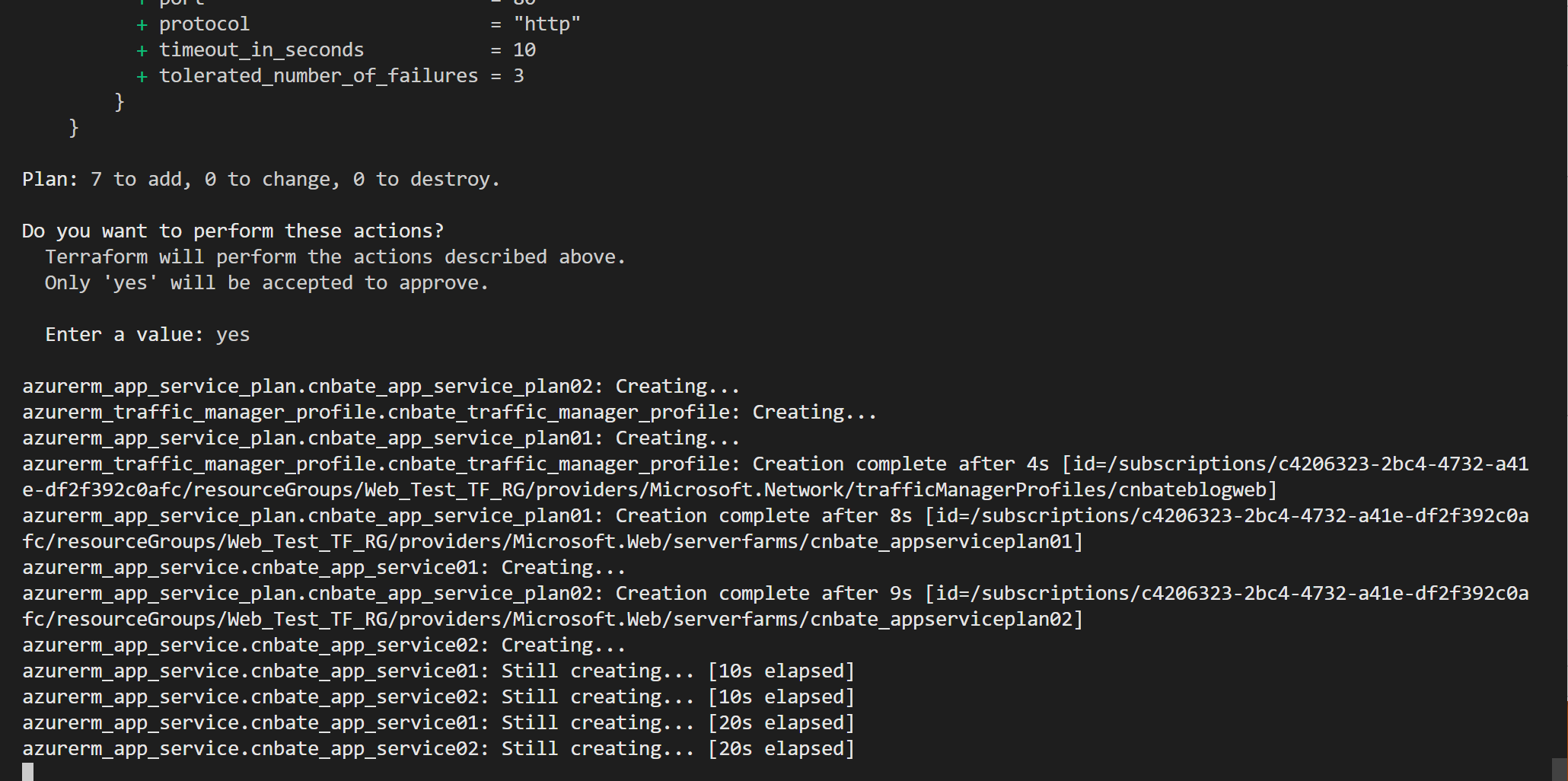LEASE STATUS：已锁定

LEASE STATE：已租用（其实可以理解为 “不可用”）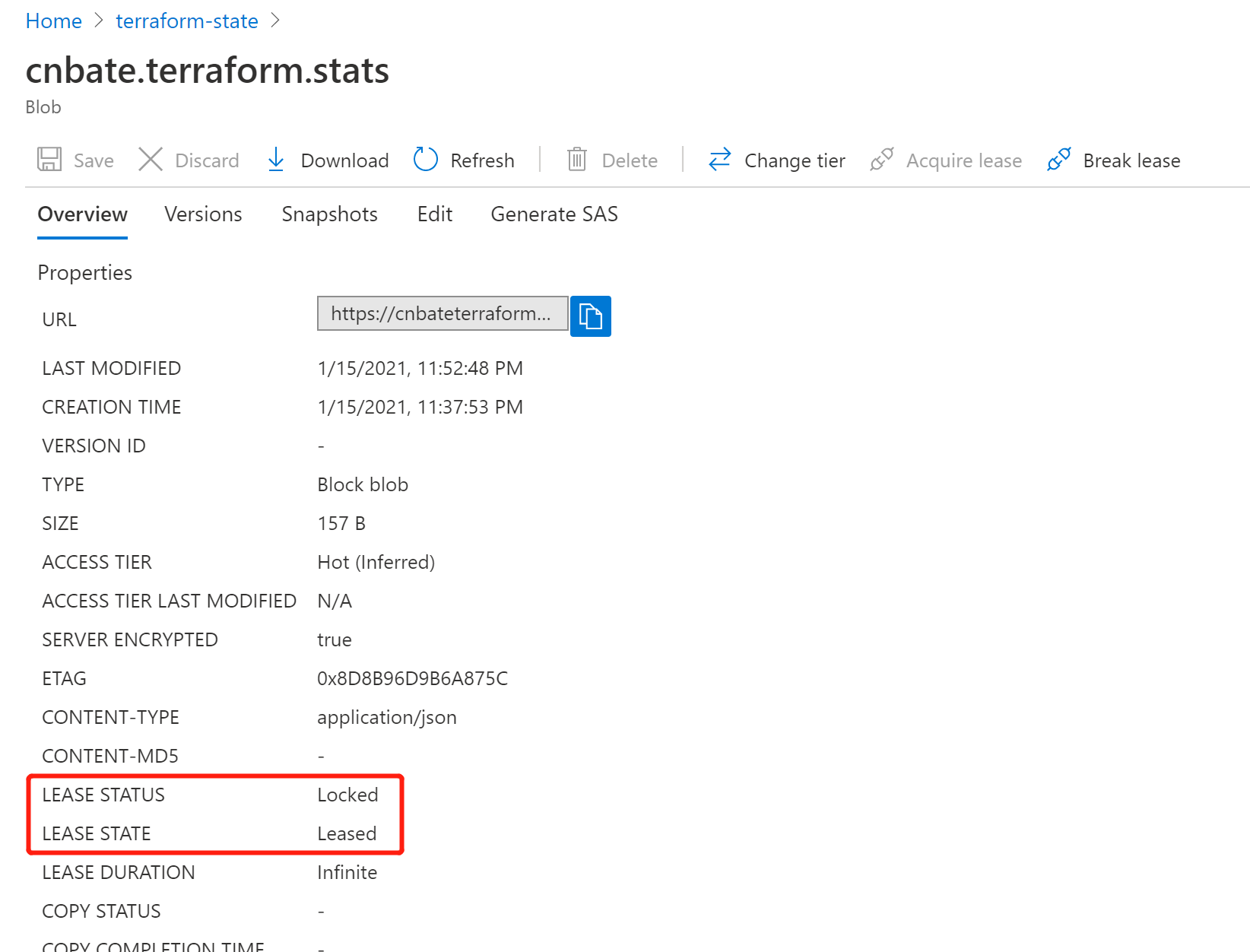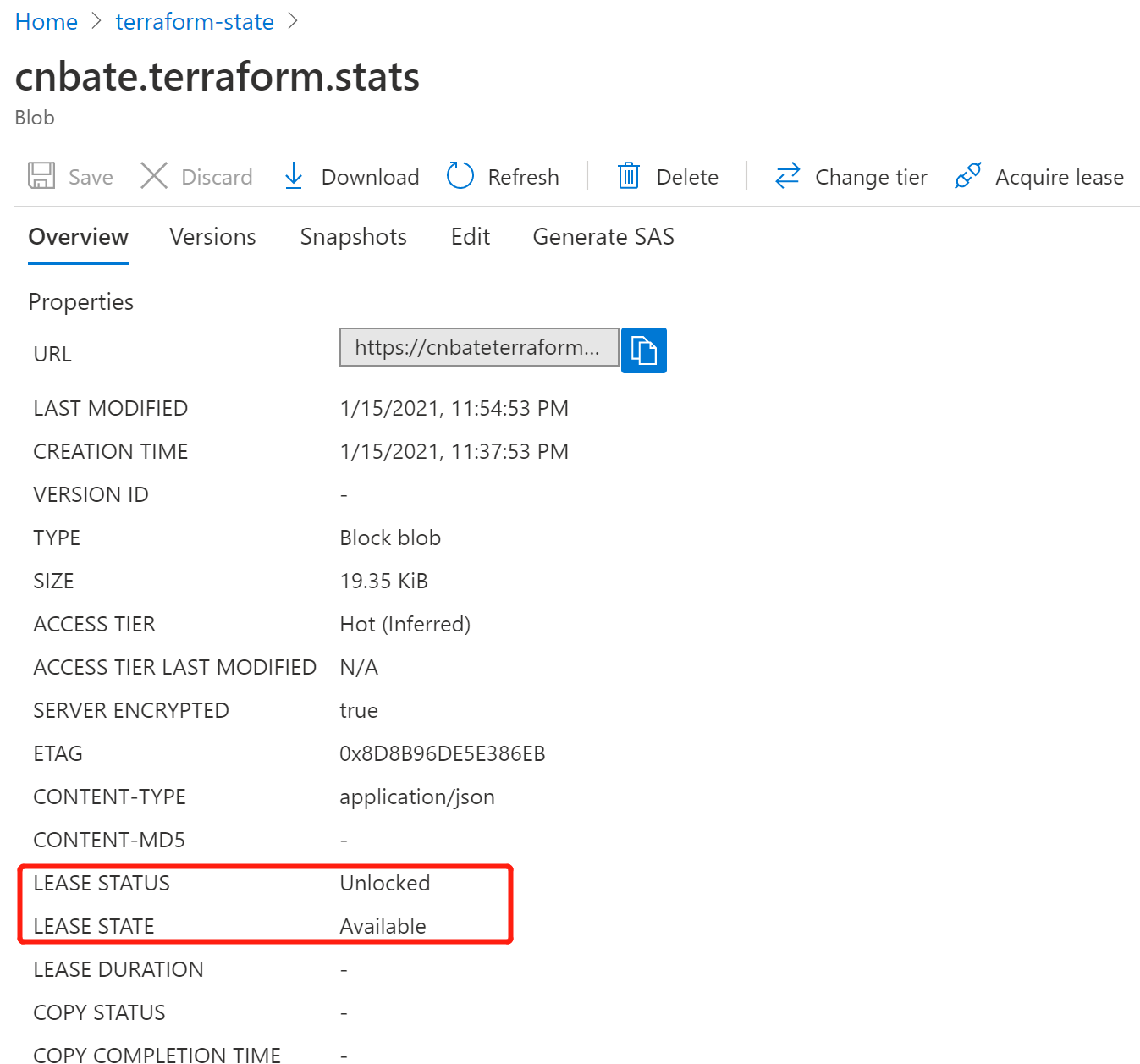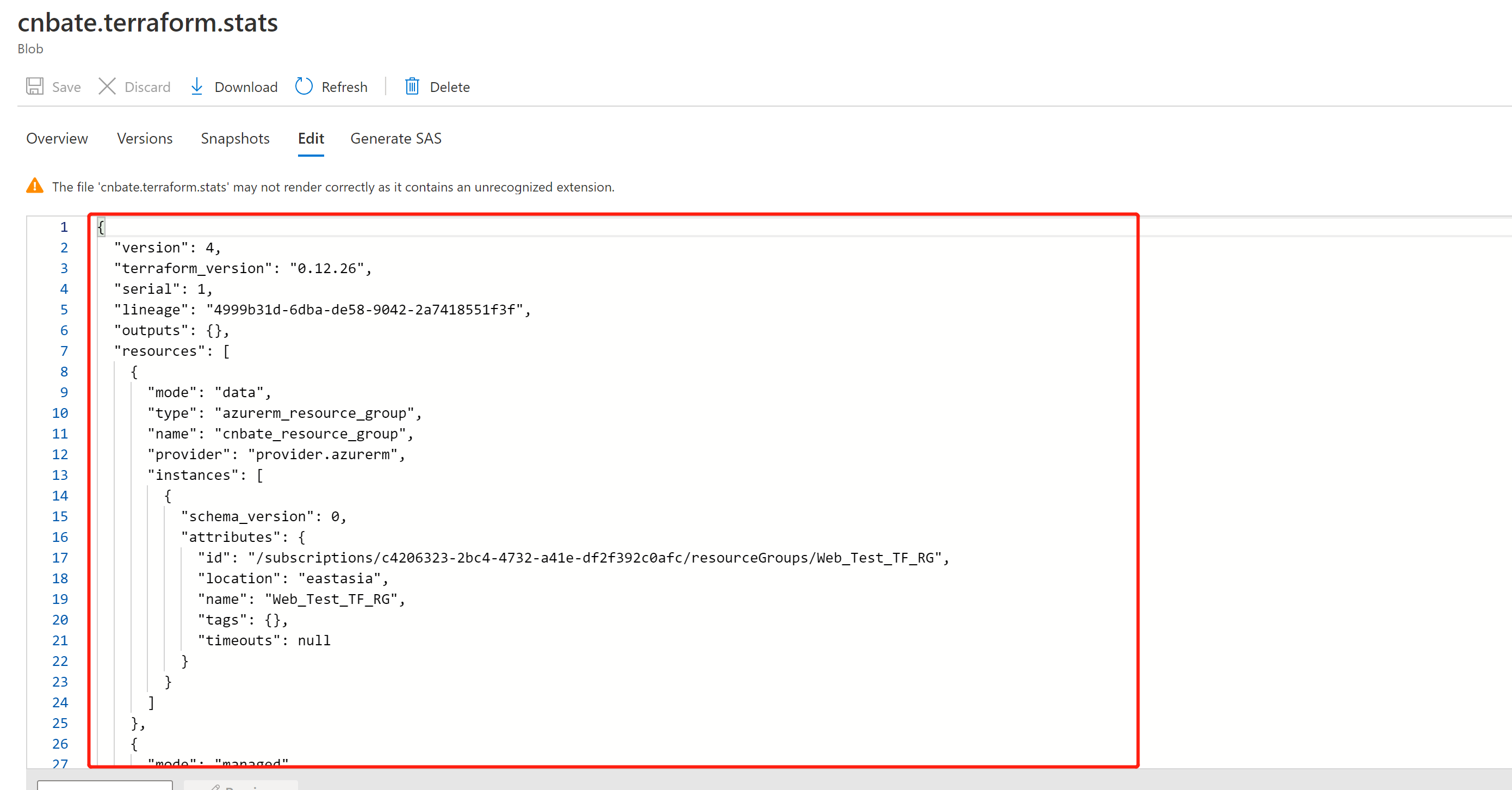ok，今天的内容就先到此。重要提醒：大家做完测试后，别忘记执行 terraform destroy (销毁部署计划)

*★,°*:.☆(￣▽￣)/\$:*.°★* 。φ(゜▽゜*)♪是✌✌✌✌✌

将状态文件进行远端存到Azure Storage Account 中，Blob 中存储的数据会在保存前进行加密处理，并且一旦配置远端存储模式后，状态文件永远不会存储在本地，这样更加方面团队之间的协作。并且远端存储带来的好处是实现了与资源定义模板管理的解耦，可以让 Terraform 状态脱离本地磁盘而存储，提升了资源状态的安全性。

Terraform_Cnbate_Traffic_Manager github：https://github.com/yunqian44/Terraform_Cnbate_Traffic_Manager

## Java中的关键字

Java中的关键字下面列出了Java关键字。这些保留字不能用于常量、变量、和任何标识符的名称。关键字都是使用小写字母的。1.访问控制类private私有的protected受保护的public公共的d...

## c++ 用类统计不及格人数_统计小课堂13

Previousreview：1)回归章节小结；这周开始计数资料统计！--------------------------------------当观察数据按...

## req.params req.query 和 req.body的区别

req.params可用于Restful的URLgetdelete传统的URLputpostreq.query可用于传统的URLgetdeletereq.body可用于Restful的URLputpo...